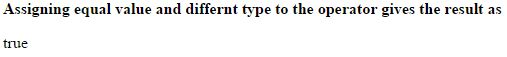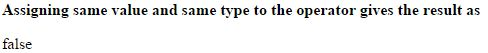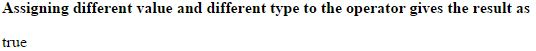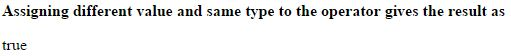# Not equal value or Not equal type (!==) operator in JavaScript

##### How to check that two operands are having not equal value or not equal type in JavaScript?

In the previous post we learnt about the Equal value and Equal type, now we shall learn the Not equal value or Not equal type.

### Not equal value or Not equal type (!==)

Not equal value or Not equal type is an comparison operator which is used to check whether the two operands are having not equal value or not equal type.

The symbolic representation of Not equal value or Not equal type is `!==`.

In this any one should be different either value or type.

NOTE:

• Same value and different type gives result 'true'.
• Same value and same type gives result 'false'.
• Diffrent value and differnt type gives result 'true'.
• Differnt value and same type gives result 'true'.

### Assigning same value and different type

```<b>Assigning equal value and differnt type to the operator gives the result as</b>
<p id="myId"></p>

<script>
var a = 30;
document.getElementById("myId").innerHTML = (a !== "30");
</script>
```

In the above code snippet we have given same value to the variable `a` and to the operator, so the result gives 'true'.

OUTPUT### Assigning same value and same type

```<b>Assigning same value and same type to the operator gives the result as</b>
<p id="myId"></p>

<script>
var a = "30";
document.getElementById("myId").innerHTML = (a !== "30");
</script>
```

Same value and same type

In the above code snippet we have assigned same value and same type to the variable `a` and to the operator, so the result gives 'false'.

OUTPUT### Assigning differnt value and different type

```<b>Assigning different value and different type to the operator gives the result as</b>
<p id="myId"></p>

<script>
var a = 30;
document.getElementById("myId").innerHTML = (a !== "20");
</script>
```

Different value and different type

In the above code snippet we have given different value and different type to the variable `a` and to the operator, so the result gives 'true'.

OUTPUT### Assigning different value and same type

```<b>Assigning different value and same type to the operator gives the result as</b>
<p id="myId"></p>

<script>
var a = 30;
document.getElementById("myId").innerHTML = (a !== 20);
</script>```

Different value and same type

In the above code snippet we have given different values of same type to the variable `a` and to the operator, so the result gives 'true'.

OUTPUTViews: 28111 | Post Order: 170